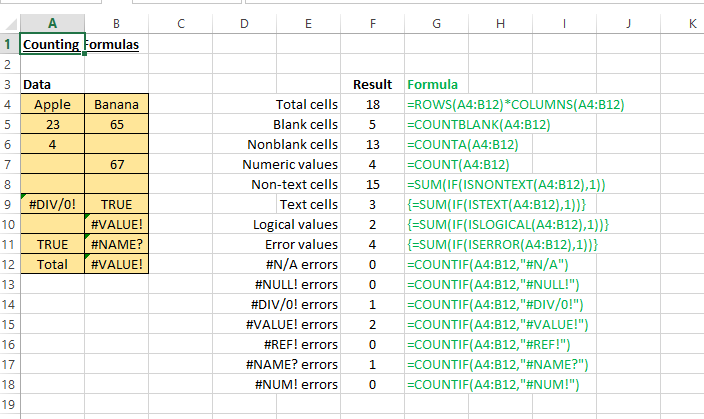# Counting Formulas in Excel

### Counting Formulas

These formulas might be very basic and simple but we find these very useful. If you are doing analysis of a huge sets of data, then this is a must-learn tutorial.

Description of Example
This example demonstrates the capability of Excel counting functions to count the number of cells in a range that meet specific criteria. The figure below shows a worksheet that uses formulas (in column F) to summarize the contents of range A4:B12. This range contains different type of information, including values, texts, logical values, errors and empty cells. In column G, it shows the relevant formula for each row.Excelcrib was founded in November 2017 by Garry, a Microsoft® Office Excel® (MS Excel) enthusiast with background in engineering. He's been using MS Excel for more than 15 years in practice with specialty in VBA.